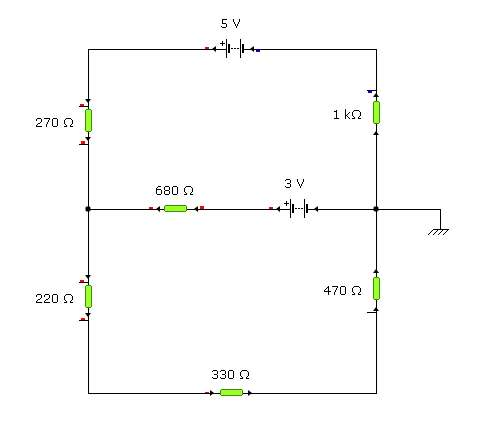# Electrical Circuit Check

## Homework StatementFor this circuit, I'm fairly confused with the extra battery. I need to find the Voltages at A (Between 5V Battery and 270 resistor), B (Between 5V Battery and 1k resistor), C (Intersection between 270, 220 and 680 resistors), E (in between 220 and 330 resistor) and F(Between 330 and 470 resistor)

I then need to find the potential difference between (using above locations): B and C, G (Between 680 resistor and 3V Battery) and A, G and F, B and G.

Finally, I need to find the currents of I1(going through point A), I2 (going into Point G) and I3 (going near point E).

2. The attempt at a solution

I wasn't really sure how to solve this mathematically due to the Extra Battery. So any good tutorial on how to do this would be appricated. I used Yenka Modelling Software to build the circuit the measure the currents / voltages. I got the following:

Potentials:

A: 3.09V
B: -1.91V
C: 2.58V
E: 2.02V
F: 1.19V

I1 = 1.91mA
I2 = 0.062mA
I3 = 2.53mA

However they look wrong :(

Thanks!

## The Attempt at a Solution

LowlyPion
Homework Helper
You treat the battery as a circuit element.

The sum of the voltages about any loop must get you back to 0.

So write two loop equations, being sure to sum the current in the top loop with the current in the bottom loop through the common path in the middle.

That means that you should end with two equations with the I1 and I2 your unknowns.

It's better to understand how to construct the loop equations than rely on modeling simulators.

Sorry I'm confused. Are there any good examples / tutorials on how to do this?

LowlyPion
Homework Helper
Here are some examples:
http://math.fullerton.edu/mathews/n2003/KirchoffMod.html [Broken]

Last edited by a moderator:
Hmm. Ok can you please check this if possible.

I1 = I2 + I3

Therefore I2 = I1 - I3?

Points A + C + E + F + D + B = 0 (Due to the voltage law?)
Points A + C + 3 + D + B = 0 (confused because of the battery...)?

Loop 1 = Top half of the circuit (270 => 680 => +3V => 1k)
Loop 2 = Bottom half of circuit (220 => 330 => 470)

Is that all correct? From there I'm not sure how to proceed. I'm pretty confused with the loops

anyone?

LowlyPion
Homework Helper
Hmm. Ok can you please check this if possible.

I1 = I2 + I3

Therefore I2 = I1 - I3?

Points A + C + E + F + D + B = 0 (Due to the voltage law?)
Points A + C + 3 + D + B = 0 (confused because of the battery...)?

Loop 1 = Top half of the circuit (270 => 680 => +3V => 1k)
Loop 2 = Bottom half of circuit (220 => 330 => 470)

Is that all correct? From there I'm not sure how to proceed. I'm pretty confused with the loops